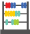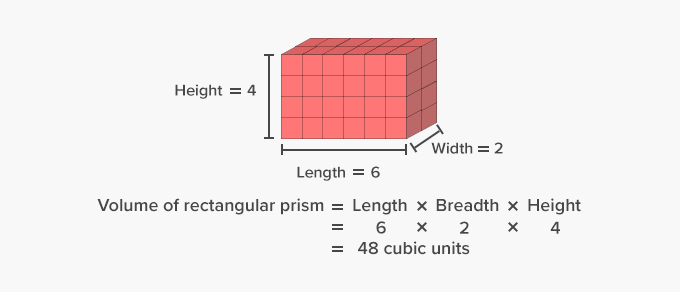# Cubic Unit - Definition with Examples

The Complete K-5 Math Learning Program Built for Your Child

• 30 Million Kids

Loved by kids and parent worldwide

• 50,000 Schools

Trusted by teachers across schools

• Comprehensive Curriculum

Aligned to Common Core

##Let's learn!

What is a cubic unit?
In geometry, cubic units can be defined as the units used to measure volume. The volume of a unit cube whose length, width and height are 1 unit each is 1 cubic unit.

Here, the rectangular prism is made up of smaller unit cubes. The length, width and height of the rectangular prism can be measured by counting the number of unit cubes. The volume is then determined in cubic units.Some examples of cubic units in metric units are cubic meters, cubic centimeters, and in customary units are cubic inches, cubic feet.

 Fun Facts All 6 faces of a cubic unit are square, and all its 12 edges measure one unit each in length.

##Let's do it!

While solving word problems on finding the volume, emphasize on the how the single unit of length, width or height turns into cubic units.

##Related math vocabulary

Won Numerous Awards & Honors